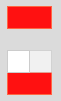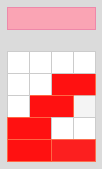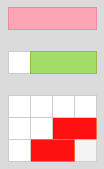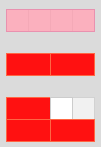#### You may also like### Tea Cups

Place the 16 different combinations of cup/saucer in this 4 by 4 arrangement so that no row or column contains more than one cup or saucer of the same colour.### Painting Cubes

Imagine you have six different colours of paint. You paint a cube using a different colour for each of the six faces. How many different cubes can be painted using the same set of six colours?### Lesser Digits

How many positive integers less than or equal to 4000 can be written down without using the digits 7, 8 or 9?

# Colour Building

##### Age 11 to 14Challenge Level

Mahdi from Mahatma Gandhi International School in India sent a full solution. This is the first part of Mahdi's solution, with some diagrams edited to show the rods being built:

Let $T_n$ define the number of ways of making a rod of length $n$ just by using red and white rods. We already know the following:

$T_1 = 1$ (there is $1$ way to make a white rod from red and white rods)

$T_2 = 2$ (there are $2$ ways to make a red rod from red and white rods)$T_3 = 3$ (there are $3$ ways to make a light green rod)$T_4 = 5$ (there are $5$ ways to make a pink rod)We can count all of the possibilities systematically making sure none is left. We observe that we start to make the $n$ length rod using one white at the left most place and cover the $n-1$ rods using red and whites (for example, put one white rod at the beginning and cover the length of the green rod using red and white rods).

The ways to cover $n-1$ length rod as defined in the beginning is $T_{n-1}$ (there were $3$ ways to make a light green rod).Next we introduce a red at the left most side and try to cover the rest of the $n-2$ remaining units with the red and the whites (in the example, put one red rod at the beginning and cover the length of the second red rod using red and white rods). The total ways of making a rod of length $n-2$ is as defined in the beginning $T_{n-2}$ (there were $2$ ways to make a red rod).

So there are $5$ ways to make a pink rod using red and white rods.

Thus we get the explicit formula for combining a rod of length $n$ using red and white as: $$T_n = T_{n+1} + T_{n+2}$$

Using this formula we can calculate $T_5, T_6, T_7, ...$

$T_5 = T_4 + T_3 = 5+3=8$
$T_6 = T_5+T_4=8+5=13$
$T_7 = T_6+T_5=13+8=21$
$T_8=T_7+T_6=21+13=34$
$T_9 = T_8 + T_7 = 34+21=55$
$T_{10} = T_9+T_8=55+34=89$ (orange rod)

You can see that $T_n=F{n-1}$ where $F_n$ is the $n^{th}$ Fibonacci number.

Mahdi's solution continues to find the definition of $T_n$ in terms of $n.$ Mahdi then considers finding lengths using white, red and green rods, or using white, red, green and pink rods, or using the first $k$ rods available. Click here to see Mahdi's full solution.